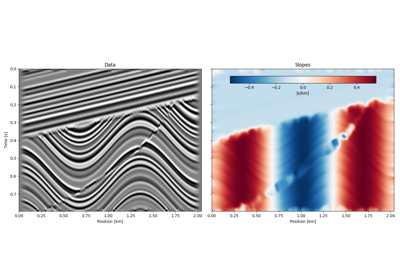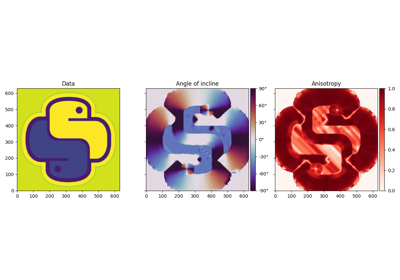# pylops.signalprocessing.Seislet#

class pylops.signalprocessing.Seislet(slopes, sampling=(1.0, 1.0), level=None, kind='haar', inv=False, dtype='float64', name='S')[source]#

Two dimensional Seislet operator.

Apply 2D-Seislet Transform to an input array given an estimate of its local slopes. In forward mode, the input array is reshaped into a two-dimensional array of size $$n_x \times n_t$$ and the transform is performed along the first (spatial) axis (see Notes for more details).

Parameters
slopesnumpy.ndarray

Slope field of size $$n_x \times n_t$$

samplingtuple, optional

Sampling steps in x- and t-axis.

levelint, optional

Number of scaling levels (must be >=0).

kindstr, optional

Basis function used for predict and update steps: haar or linear.

invint, optional

Apply inverse transform when invoking the adjoint (True) or not (False). Note that in some scenario it may be more appropriate to use the exact inverse as adjoint of the Seislet operator even if this is not an orthogonal operator and the dot-test would not be satisfied (see Notes for details). Otherwise, the user can access the inverse directly as method of this class.

dtypestr, optional

Type of elements in input array.

namestr, optional

New in version 2.0.0.

Name of operator (to be used by pylops.utils.describe.describe)

Raises
NotImplementedError

If kind is different from haar or linear

ValueError

If sampling has more or less than two elements.

Notes

The Seislet transform  is implemented using the lifting scheme.

In its simplest form (i.e., corresponding to the Haar basis function for the Wavelet transform) the input dataset is separated into even ($$\mathbf{e}$$) and odd ($$\mathbf{o}$$) traces. Even traces are used to forward predict the odd traces using local slopes and the new odd traces (also referred to as residual) is defined as:

$\mathbf{o}^{i+1} = \mathbf{r}^i = \mathbf{o}^i - P(\mathbf{e}^i)$

where $$P = P^+$$ is the slope-based forward prediction operator (which is here implemented as a sinc-based resampling). The residual is then updated and summed to the even traces to obtain the new even traces (also referred to as coarse representation):

$\mathbf{e}^{i+1} = \mathbf{c}^i = \mathbf{e}^i + U(\mathbf{o}^{i+1})$

where $$U = P^- / 2$$ is the update operator which performs a slope-based backward prediction. At this point $$\mathbf{e}^{i+1}$$ becomes the new data and the procedure is repeated level times (at maximum until $$\mathbf{e}^{i+1}$$ is a single trace. The Seislet transform is effectively composed of all residuals and the coarsest data representation.

In the inverse transform the two operations are reverted. Starting from the coarsest scale data representation $$\mathbf{c}$$ and residual $$\mathbf{r}$$, the even and odd parts of the previous scale are reconstructed as:

$\mathbf{e}^i = \mathbf{c}^i - U(\mathbf{r}^i) = \mathbf{e}^{i+1} - U(\mathbf{o}^{i+1})$

and:

$\mathbf{o}^i = \mathbf{r}^i + P(\mathbf{e}^i) = \mathbf{o}^{i+1} + P(\mathbf{e}^i)$

A new data is formed by interleaving $$\mathbf{e}^i$$ and $$\mathbf{o}^i$$ and the procedure repeated until the new data as the same number of traces as the original one.

Finally the adjoint operator can be easily derived by writing the lifting scheme in a matricial form:

$\begin{split}\begin{bmatrix} \mathbf{r}_1 \\ \mathbf{r}_2 \\ \vdots \\ \mathbf{r}_N \\ \mathbf{c}_1 \\ \mathbf{c}_2 \\ \vdots \\ \mathbf{c}_N \end{bmatrix} = \begin{bmatrix} \mathbf{I} & \mathbf{0} & \ldots & \mathbf{0} & -\mathbf{P} & \mathbf{0} & \ldots & \mathbf{0} \\ \mathbf{0} & \mathbf{I} & \ldots & \mathbf{0} & \mathbf{0} & -\mathbf{P} & \ldots & \mathbf{0} \\ \vdots & \vdots & \ddots & \vdots & \vdots & \vdots & \ddots & \vdots \\ \mathbf{0} & \mathbf{0} & \ldots & \mathbf{I} & \mathbf{0} & \mathbf{0} & \ldots & -\mathbf{P} \\ \mathbf{U} & \mathbf{0} & \ldots & \mathbf{0} & \mathbf{I}-\mathbf{UP} & \mathbf{0} & \ldots & \mathbf{0} \\ \mathbf{0} & \mathbf{U} & \ldots & \mathbf{0} & \mathbf{0} & \mathbf{I}-\mathbf{UP} & \ldots & \mathbf{0} \\ \vdots & \vdots & \ddots & \vdots & \vdots & \vdots & \ddots & \vdots \\ \mathbf{0} & \mathbf{0} & \ldots & \mathbf{U} & \mathbf{0} & \mathbf{0} & \ldots & \mathbf{I}-\mathbf{UP} \end{bmatrix} \begin{bmatrix} \mathbf{o}_1 \\ \mathbf{o}_2 \\ \vdots \\ \mathbf{o}_N \\ \mathbf{e}_1 \\ \mathbf{e}_2 \\ \vdots \\ \mathbf{e}_N \end{bmatrix}\end{split}$

$\begin{split}\begin{bmatrix} \mathbf{o}_1 \\ \mathbf{o}_2 \\ \vdots \\ \mathbf{o}_N \\ \mathbf{e}_1 \\ \mathbf{e}_2 \\ \vdots \\ \mathbf{e}_N \end{bmatrix} = \begin{bmatrix} \mathbf{I} & \mathbf{0} & \ldots & \mathbf{0} & -\mathbf{U^T} & \mathbf{0} & \ldots & \mathbf{0} \\ \mathbf{0} & \mathbf{I} & \ldots & \mathbf{0} & \mathbf{0} & -\mathbf{U^T} & \ldots & \mathbf{0} \\ \vdots & \vdots & \ddots & \vdots & \vdots & \vdots & \ddots & \vdots \\ \mathbf{0} & \mathbf{0} & \ldots & \mathbf{I} & \mathbf{0} & \mathbf{0} & \ldots & -\mathbf{U^T} \\ \mathbf{P^T} & \mathbf{0} & \ldots & \mathbf{0} & \mathbf{I}-\mathbf{P^T U^T} & \mathbf{0} & \ldots & \mathbf{0} \\ \mathbf{0} & \mathbf{P^T} & \ldots & \mathbf{0} & \mathbf{0} & \mathbf{I}-\mathbf{P^T U^T} & \ldots & \mathbf{0} \\ \vdots & \vdots & \ddots & \vdots & \vdots & \vdots & \ddots & \vdots \\ \mathbf{0} & \mathbf{0} & \ldots & \mathbf{P^T} & \mathbf{0} & \mathbf{0} & \ldots & \mathbf{I}-\mathbf{P^T U^T} \end{bmatrix} \begin{bmatrix} \mathbf{r}_1 \\ \mathbf{r}_2 \\ \vdots \\ \mathbf{r}_N \\ \mathbf{c}_1 \\ \mathbf{c}_2 \\ \vdots \\ \mathbf{c}_N \end{bmatrix}\end{split}$

which can be written more easily in the following two steps:

$\mathbf{o} = \mathbf{r} + \mathbf{U}^H\mathbf{c}$

and:

$\mathbf{e} = \mathbf{c} - \mathbf{P}^H(\mathbf{r} + \mathbf{U}^H(\mathbf{c})) = \mathbf{c} - \mathbf{P}^H\mathbf{o}$

Similar derivations follow for more complex wavelet bases.

1

Fomel, S., Liu, Y., “Seislet transform and seislet frame”, Geophysics, 75, no. 3, V25-V38. 2010.

Attributes
shapetuple

Operator shape

explicitbool

Operator contains a matrix that can be solved explicitly (True) or not (False)

Methods

 __init__(slopes[, sampling, level, kind, ...]) adjoint() apply_columns(cols) Apply subset of columns of operator cond([uselobpcg]) Condition number of linear operator. conj() Complex conjugate operator div(y[, niter, densesolver]) Solve the linear problem $$\mathbf{y}=\mathbf{A}\mathbf{x}$$. dot(x) Matrix-matrix or matrix-vector multiplication. eigs([neigs, symmetric, niter, uselobpcg]) Most significant eigenvalues of linear operator. inverse(x) matmat(X) Matrix-matrix multiplication. matvec(x) Matrix-vector multiplication. reset_count() Reset counters rmatmat(X) Matrix-matrix multiplication. rmatvec(x) Adjoint matrix-vector multiplication. todense([backend]) Return dense matrix. toimag([forw, adj]) Imag operator toreal([forw, adj]) Real operator tosparse() Return sparse matrix. trace([neval, method, backend]) Trace of linear operator. transpose()

## Examples using pylops.signalprocessing.Seislet#Seislet transform

Seislet transformSlope estimation via Structure Tensor algorithm

Slope estimation via Structure Tensor algorithm